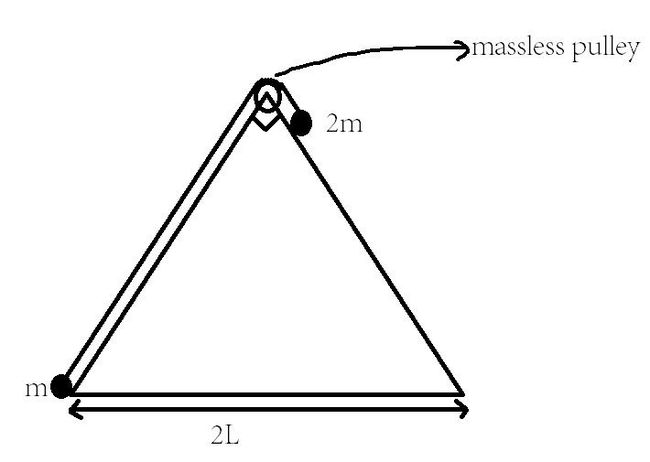# System of Particles on a wedge!A right angled isosceles triangular wedge of mass $m$ is placed on a smooth horizontal surface. Two point like masses $m$ & $2m$, tied to each other by a thread, are placed on the wedge. Friction & masses of the thread & pulley are negligible. The bodies are released at $t=0$. The distance moved by the wedge until the "instant $2m$ reaches the bottom of wedge" is

×